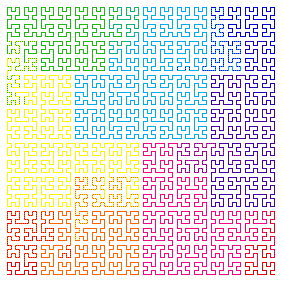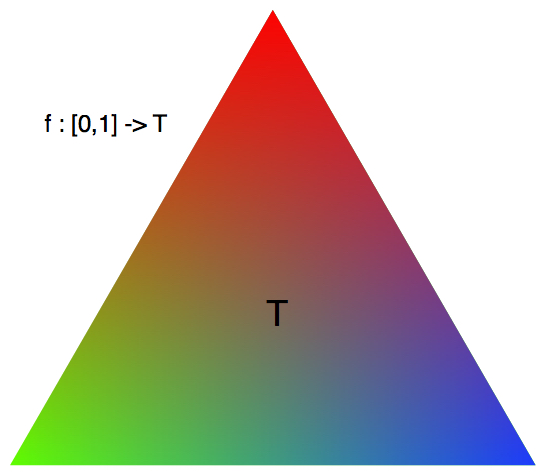# Continuous Coloring

Geometry Level 5Let $T$ be an equilateral triangle of side length $1$, whose vertices are located at $(0,0), (1,0)$ and $\left(\frac{1}{2}, \frac{\sqrt{3}}{2}\right)$. Let $(f_i), i=1,2,3, \ldots$ be continuous functions such that, $f_i : [0,1] \to T$ i.e., each point in $[0,1]$ is mapped to some point in the triangle $T$ by each function $f_i$.

Think of each $f_i$ as a pen with color $i$. So that each $f_i$ colors its range (which is some portion of the triangle $T$ ) with the color $i$. E.g., if $f_1(1/3)=(\frac{1}{2},\frac{1}{2})$, this means that the point $(\frac{1}{2},\frac{1}{2})$ is colored '1' by the function $f_1$.

Find the minimum number of continuous functions $f_i$ needed to color the entire triangle.

×